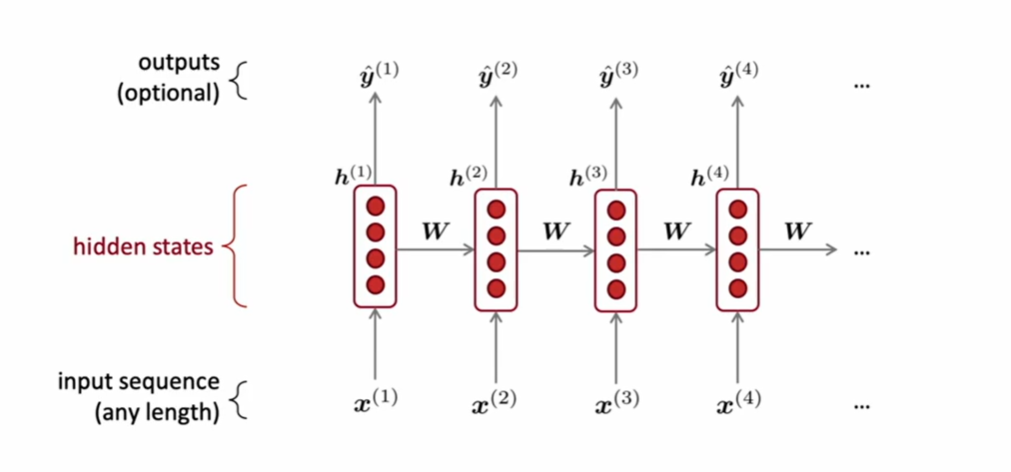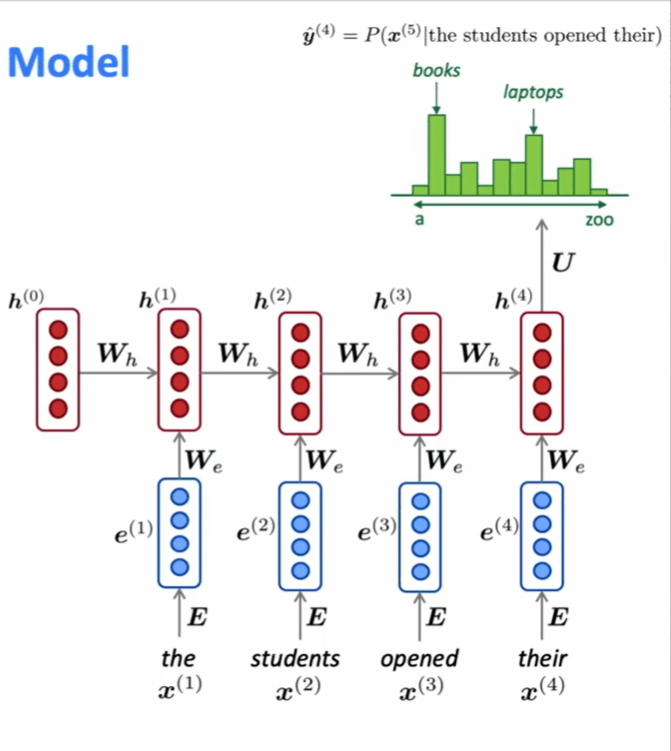# Course 6 - Language Models and RNNs

## Language Modeling

is the task of predicting what word comes next.

also, assigns probability to a piece of text

### n-gram Language Models

#### Definition

A n-gram is a chunk of n consecutive words

#### Idea

Collect statistics about how frequent different n-grams are, and use these to predict next word.

#### Sparity Problem 1: what if “students opened their w“ never occurred in data

Solution-Smoothing: Add small delta to the count for every w

#### Sparity Problem2: what if “students opened their” never occurred in data?

Solution-backoff: Just condition on “opened their” instead

## How to build a neural Language Model - Recurrent Neural Networks

### Recurrent Neural Networks(RNN)Core idea: Apply the same weight W repeatedly.• Can process any length input
• use information from many steps back in theory
• Model size doesn’t increase for longer input
• Same weights applied on every steps.

• slow computation
• difficult to access information from many steps back practically

#### Training a RNN Language Model

• Get a big corpus of text which is a sequence of word $x^{1}, \cdots, x^{T}$

• Feed into RNN-LM

• Loss function on step t is cross-entropy

• Average this to get overall loss for entire training set

• However, computing loss and gradients across entire corpus is too expensive. In practice, consider $x^{1}, \cdots, x^{T}$ as a sentence (or a document)

• Instead, using SGD to compute loss $J(\theta)$ for a sentence, compute gradients and update weights. Repeat.

#### Backpropagation for RNNs

Questions: derivative of $J^{(t)}(\theta)$ w.r.t the repeated weight matrix

#### Generating text with a RNN Language Model.

repeated sampling

#### Evaluating Language Models: Perplexity

lower perplexity is better!

#### Why should we care about Language Modeling?

Language Modeling is a benchmark task that helps us measure our progress on understanding language.